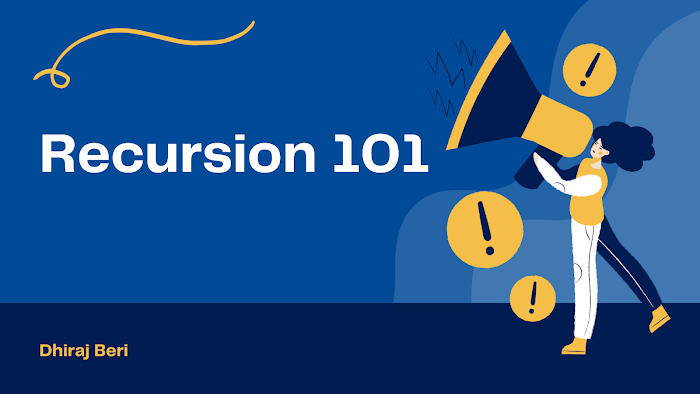# What is Recursion? Types of Recursion.

Hello guys, In this tutorial we are going to see what recursion is?

The process in which a function calls itself directly or indirectly is called recursion and the corresponding function is called a recursive function. Using a recursive algorithm, certain problems can be solved quite easily. And it is one of the most important topics of this course.

So, first of all, let's see the types of recursion,

# Types of recursion

1. Tail - Linear

3. Tree

4. Indirect

5. Nested

So, let's see them one by one with examples. But first we need to understand some terms like calling time and returning time. You can understand it by the code snippet.

`void fun(int n){    if(n>0)    {        --statements-- //calling time        fun(n-1)+n; //return time        --statements-- //return time    }}`

Tail Recursion
`#include <stdio.h>void fun(int n){ if(n>0) {   printf("%d ",n);   fun(n-1); }}int main() { int x=3; fun(3); return 0;}`

`#include <stdio.h>void fun(int n){ if(n>0) {   fun(n-1);   printf("%d ",n); }}int main() { int x=3; fun(3); return 0;}`

Static Global
`#include <stdio.h>int fun(int n){ static int x=0; if(n>0) {   x++;   return fun(n-1)+x; //return time } return 0;}int main() { int a=5; printf("%d ",fun(a));}`

Tree Recursion
`#include <stdio.h>void fun(int n){ if(n>0) {   printf("%d ",n);   fun(n-1);   fun(n-1); }}int main() { int x=3; fun(3); return 0;}`

Indirect Recursion
`#include <stdio.h>void fun2(int n);void fun1(int n){ if(n>0) {   printf("%d ",n);   fun2(n-1); }}void fun2(int n){ if(n>0) {   printf("%d ",n);   fun1(n/2); }}int main() { fun1(20); return 0;}`

Nested Recursion
`#include <stdio.h>int fun(int n){ if(n>100) {   return n-10; } else {   return fun(fun(n+11)); }}int main(void) { printf("%d ",fun(95)); return 0;}`

# Conclusion

In this tutorial, we all learn that what is recursion and it's type. In the next blog we will look into some problems and try to solve them using recursion. What problems can be solved using recursion?